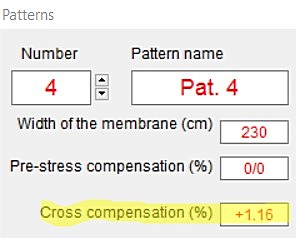The common way of patterning of a tensile membrane is to break down to triangles which are always flat (see the below figure). This series of adjacent triangles is called as pattern.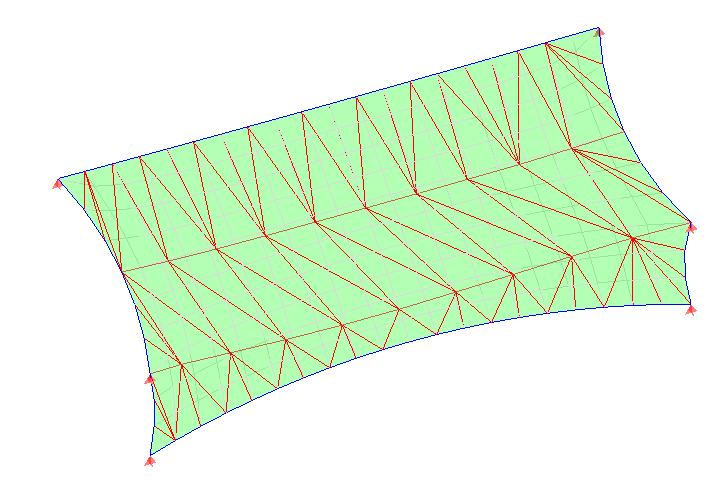Triangles on the membrane

It is obvious that the triangles are defined by the sides, and these are straight segments while the membrane surface is curved (almost always double curvature). Therefore triangles are always smaller than the area representing membrane. The program WinTess has a tool to verify that the distance between the triangle (its centroid) and the surface of the membrane is small and, therefore, the error produced is also a small.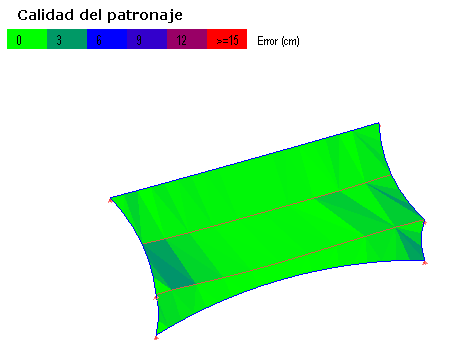Checking quality of a pattern

However, there are cases where due to a pronounced double curvature it is practically impossible to make a pattern that has good quality using triangles of normal dimensions. Good quality means that the triangles are close to the membrane surface.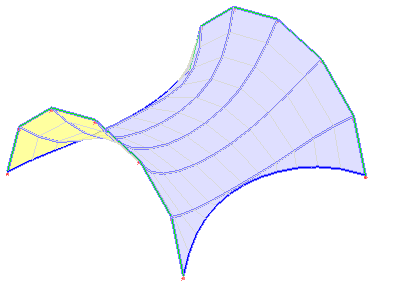Double arch with very pronounced curvature

Let’s suppose the membrane of the previous image. A very pronounced double arch structure with 6 patterns, from arch to arch.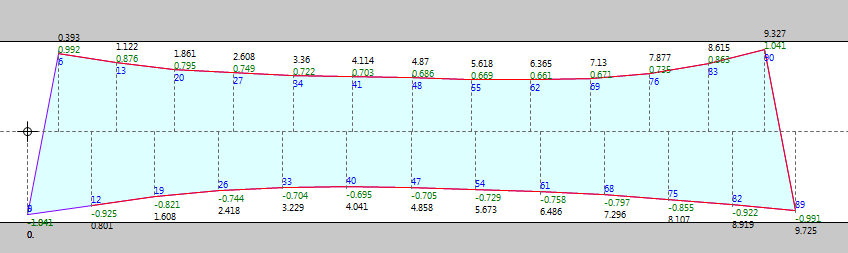Central pattern

We see that the central pattern (one of the two) has a normal appearance. We also see that the long edges form a gentle curve, therefore we need to assume that the errors that may exist in the total length of these sides is going to be small. The proportion between the length of the real curve and the straight line (the side of the triangle) is a function of curvature. In the example we are discussing, the width of arches at ends is 8 m and the height of its center point is 4 m. Therefore the arc opening is 180° = Pi rad. Each pattern (there are six) covers 180° / 6 = 30° = Pi / 6 rad. If we do necessary geometric calculations we will see that the relationship between an arc of 30 degrees and your rope is in:
`arch / rope= 0,5236 / 0,5176 = 1.0116`
Therefore it is necessary to increase the cross dimensions by a unitary value of 0.0116
`0.0116 = 1.16 %`
WinTes3 allows you to make this cross compensation automatically through the corresponding checkbox. Let’s remember that we can compensate independently every pattern if the cross curvature is different in each of them. If we are to compensate them all alike, we first select All from menu and then enter the value of compensation as per cent.